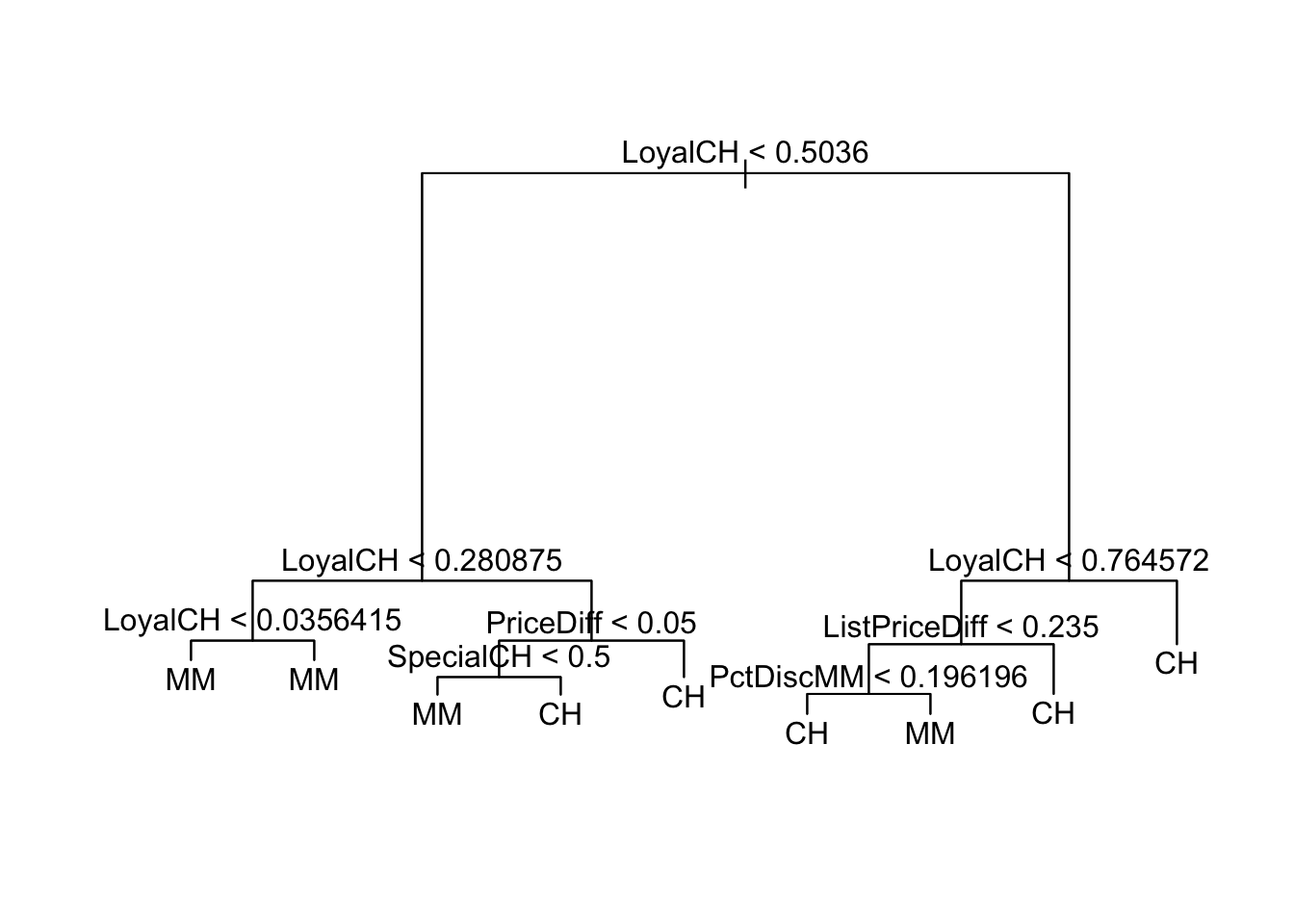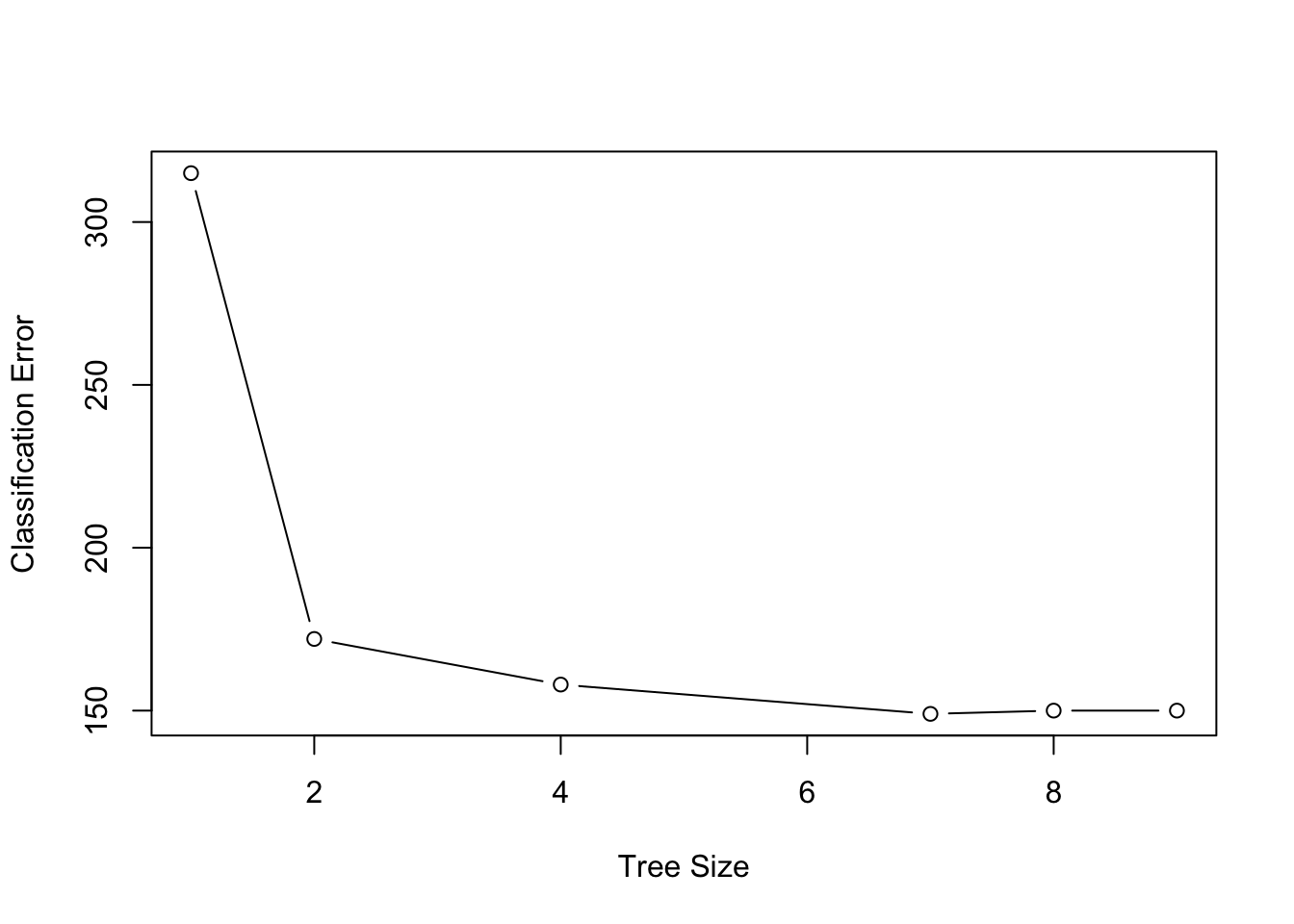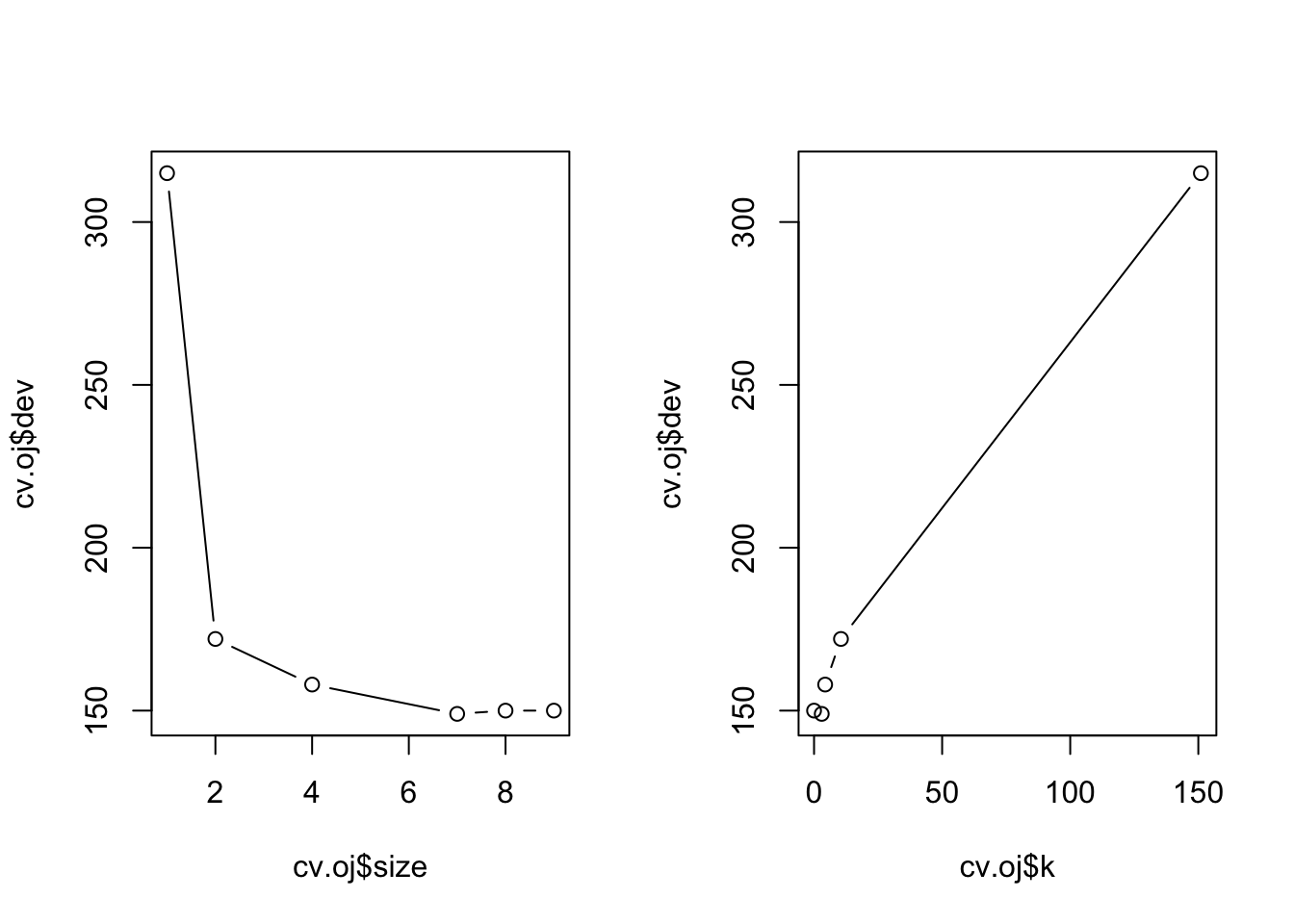ISLR Home

# Question

p334

This problem involves the OJ data set which is part of the ISLR package.

1. Create a training set containing a random sample of 800 obser- vations, and a test set containing the remaining observations.

2. Fit a tree to the training data, with Purchase as the response and the other variables as predictors. Use the summary() function to produce summary statistics about the tree, and describe the results obtained. What is the training error rate? How many terminal nodes does the tree have?

3. Type in the name of the tree object in order to get a detailed text output. Pick one of the terminal nodes, and interpret the information displayed.

4. Create a plot of the tree, and interpret the results.

5. Predict the response on the test data, and produce a confusion matrix comparing the test labels to the predicted test labels. What is the test error rate?

6. Apply the cv.tree() function to the training set in order to determine the optimal tree size.

7. Produce a plot with tree size on the x-axis and cross-validated classification error rate on the y-axis.

8. Which tree size corresponds to the lowest cross-validated classi- fication error rate?

9. Produce a pruned tree corresponding to the optimal tree size obtained using cross-validation. If cross-validation does not lead to selection of a pruned tree, then create a pruned tree with five terminal nodes.

10. Compare the training error rates between the pruned and un- pruned trees. Which is higher?

11. Compare the test error rates between the pruned and unpruned trees. Which is higher?

``````library(ISLR)
library(tree)``````

Similar to Lab: 8.3.1 Fitting Classification Trees

target = OJ\$Purchase

Purchase: A factor with levels CH and MM indicating whether the customer purchased Citrus Hill or Minute Maid Orange Juice

# Overview

• Build Tree with Training Data: tree.oj
• Predict Training Data Error on unpruned tree
• Prune Tree: prune.oj
• Predict Training Data Error on pruned tree
• Predict Test Data Error on unpruned tree
• Predict Test Data Error on pruned tree

# 9a

Create a training set containing a random sample of 800 observations, and a test set containing the remaining observations.

``dim(OJ)``
``##  1070   18``
``````set.seed(1)
train = sample(1:nrow(OJ), 800)

# Don't actually use these ???
oj.train = OJ[train,]
oj.test = OJ[-train,]
oj.train.y = OJ[train,"Purchase"]
oj.test.y = OJ[-train,"Purchase"]``````

# 9b Fit Tree to Training

Fit a tree to the training data, with Purchase as the response and the other variables as predictors. Use the summary() function to produce summary statistics about the tree, and describe the results obtained. What is the training error rate? How many terminal nodes does the tree have?

``````tree.oj = tree(Purchase ~ ., OJ, subset=train)
summary(tree.oj)``````
``````##
## Classification tree:
## tree(formula = Purchase ~ ., data = OJ, subset = train)
## Variables actually used in tree construction:
##  "LoyalCH"       "PriceDiff"     "SpecialCH"     "ListPriceDiff"
##  "PctDiscMM"
## Number of terminal nodes:  9
## Residual mean deviance:  0.7432 = 587.8 / 791
## Misclassification error rate: 0.1588 = 127 / 800``````

Uses only 5 predictors to split the tree.

## Training error rate

Training error rate: Misclassification rate: 15.88% (p324)

## How many terminal nodes does the tree have?

terminal nodes: 9

# 9c

Type in the name of the tree object in order to get a detailed text output. Pick one of the terminal nodes, and interpret the information displayed.

• denotes terminal node
``tree.oj``
``````## node), split, n, deviance, yval, (yprob)
##       * denotes terminal node
##
##  1) root 800 1073.00 CH ( 0.60625 0.39375 )
##    2) LoyalCH < 0.5036 365  441.60 MM ( 0.29315 0.70685 )
##      4) LoyalCH < 0.280875 177  140.50 MM ( 0.13559 0.86441 )
##        8) LoyalCH < 0.0356415 59   10.14 MM ( 0.01695 0.98305 ) *
##        9) LoyalCH > 0.0356415 118  116.40 MM ( 0.19492 0.80508 ) *
##      5) LoyalCH > 0.280875 188  258.00 MM ( 0.44149 0.55851 )
##       10) PriceDiff < 0.05 79   84.79 MM ( 0.22785 0.77215 )
##         20) SpecialCH < 0.5 64   51.98 MM ( 0.14062 0.85938 ) *
##         21) SpecialCH > 0.5 15   20.19 CH ( 0.60000 0.40000 ) *
##       11) PriceDiff > 0.05 109  147.00 CH ( 0.59633 0.40367 ) *
##    3) LoyalCH > 0.5036 435  337.90 CH ( 0.86897 0.13103 )
##      6) LoyalCH < 0.764572 174  201.00 CH ( 0.73563 0.26437 )
##       12) ListPriceDiff < 0.235 72   99.81 MM ( 0.50000 0.50000 )
##         24) PctDiscMM < 0.196196 55   73.14 CH ( 0.61818 0.38182 ) *
##         25) PctDiscMM > 0.196196 17   12.32 MM ( 0.11765 0.88235 ) *
##       13) ListPriceDiff > 0.235 102   65.43 CH ( 0.90196 0.09804 ) *
##      7) LoyalCH > 0.764572 261   91.20 CH ( 0.95785 0.04215 ) *``````

## Interpret one terminal node

``````"8) LoyalCH < 0.0356415 59   10.14 MM ( 0.01695 0.98305 ) *"
"9) LoyalCH > 0.0356415 118  116.40 MM ( 0.19492 0.80508 ) *"``````

### Branch 8

``````59 - Number observations in that branch
10.14 - Deviance
MM - Predicted class
( 0.01695 0.98305 ) - (Prob CH, Prob MM)``````

This branching (8) looks redundant because MM is always chosen.

### Branch 9

118 - Number observations in that branch

# 9d Plot Unpruned Tree

Create a plot of the tree, and interpret the results.

``````{plot(tree.oj)
text(tree.oj, pretty=0)
}``````## Interpretation of Results

9 terminal nodes. At least least 1 redundant node.

# 9e Predict Test Data from Unpruned

Predict the response on the test data, and produce a confusion matrix comparing the test labels to the predicted test labels. What is the test error rate?

Predict Test Response SKIP

``tree.pred = predict(tree.oj, oj.test, type="class")``

Calculate Error Rate of Training Data

``````yhat = predict(tree.oj, newdata = OJ[-train ,], type = "class")
oj.test.y = OJ[-train, "Purchase"] # Y target vector``````

## Confusion Matrix

``table(yhat, oj.test.y)``
``````##     oj.test.y
## yhat  CH  MM
##   CH 160  38
##   MM   8  64``````

Calculate Test Error Rate for unpruned tree

``(160+64)/270``
``##  0.8296296``
``summary(OJ\$Purchase)``
``````##  CH  MM
## 653 417``````

# 9f Find Optimal Prune Size

Apply the cv.tree() function to the training set in order to determine the optimal tree size.

``````cv.oj=cv.tree(tree.oj, FUN=prune.misclass)
summary(cv.oj)``````
``````##        Length Class  Mode
## size   6      -none- numeric
## dev    6      -none- numeric
## k      6      -none- numeric
## method 1      -none- character``````
``names(cv.oj)``
``##  "size"   "dev"    "k"      "method"``
``cv.oj``
``````## \$size
##  9 8 7 4 2 1
##
## \$dev
##  150 150 149 158 172 315
##
## \$k
##        -Inf   0.000000   3.000000   4.333333  10.500000 151.000000
##
## \$method
##  "misclass"
##
## attr(,"class")
##  "prune"         "tree.sequence"``````

7 is the optimal number of terminal nodes. Small misclassification error with 149 (\$dev=149)

# 9g Plot Tree Size vs Classification Error Rate

Produce a plot with tree size on the x-axis and cross-validated classification error rate on the y-axis.

``plot(cv.oj\$size, cv.oj\$dev, type='b', xlab="Tree Size", ylab="Classification Error")``Plot the error rate as a function of both size and k. (p326) Type=“b” means plot both “p” points, “l” lines

``````par(mfrow=c(1,2))
plot(cv.oj\$size, cv.oj\$dev, type="b")
plot(cv.oj\$k,cv.oj\$dev, type="b")``````# 9h

Which tree size corresponds to the lowest cross-validated classification error rate?

7 is the optimal.

# 9i Prune Training Tree

Produce a pruned tree corresponding to the optimal tree size obtained using cross-validation. If cross-validation does not lead to selection of a pruned tree, then create a pruned tree with five terminal nodes.

``````prune.oj=prune.tree(tree.oj, best=7)
{plot(prune.oj)
text(prune.oj, pretty=0)
}``````